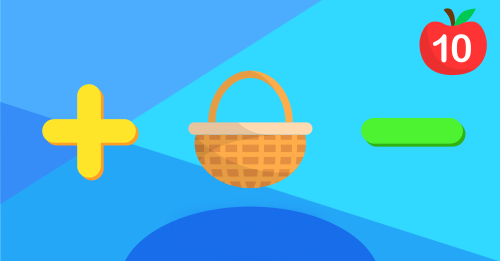Let's count these raspberries together!Can you tell the order of these animals?Which one is longer?Can you count these cupcakes?Can you tell the color of these trucks?Let's do some simple counting.Can you count these pizza?Can you identify these numbers?Can you count these ladybugs?Can you find the correct capital letter?Which one is the animal we want?Can you identify numbers up to 10?Which one is the vegetable that we want?Let's count these peanuts and nuts together!How many vehicles are there?Which one is the food that we want?Which one is longer?Let's count these kiwis together!Can you count these melons?Let's do some simple calculations.